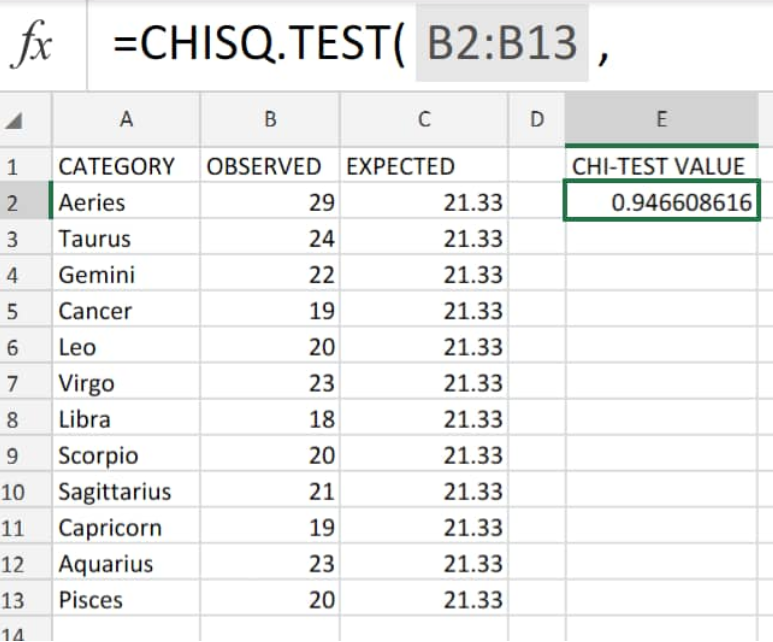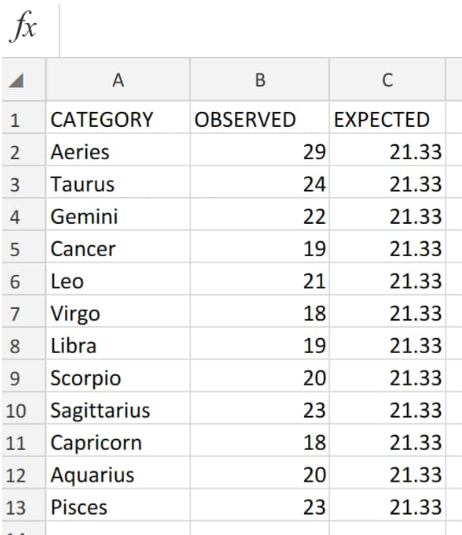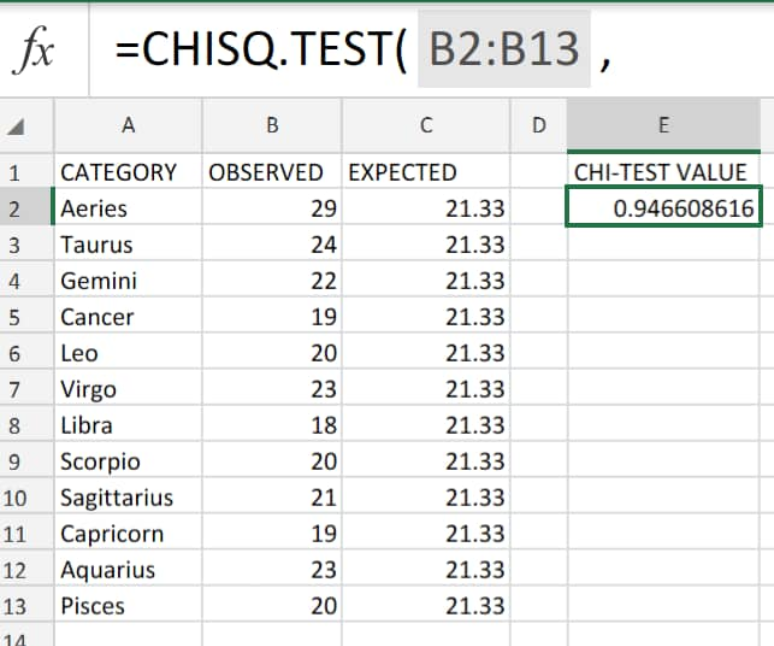Get instant live expert help with Excel or Google Sheets“My Excelchat expert helped me in less than 20 minutes, saving me what would have been 5 hours of work!”

#### Post your problem and you'll get expert help in seconds

Your message must be at least 40 characters
Our professional experts are available now. Your privacy is guaranteed.

# How to use the Chi-square test in Excel

The chi square test allows us to determine whether two different sets of data are existing independently.

There are many statistical distributions programmed into Microsoft Excel; the chi square tests are examples of such distributions.Figure 1. of Chitest in Excel

## Generic Formula

`CHISQ.TEST=(actual_range,expected_range)`

The syntax for the chisq.test formula comprises of the following arguments:

-Actual_range or R1 (required) The data range which contains the observations we would like to test against our expected values.

-Expected_range or R2 (required) The data range containing the product ratio of the row and column totals against the grand total.

## How to do Chi Square Test

We can extend the chisq.test function to support ranges which consist of multiple columns and rows.

The R1 and R2 ranges should have the same shape and size and must contain numeric values only.

Let’s assume we have a data range of values containing the results of a survey conducted among several adults having different horoscopic events, and another range of expected values which we are required to run a chi-square test for their degree of independence.

1. We start by arranging our data sets in separate columns of our worksheet;Figure 2. Of Data Sets for Chitest

1. The chisq.test formula we will enter into cell E5 of the above worksheet example is as follows:

`CHISQ.TEST(B2:B13, C2:C13)` – this should test for the degree of freedom of the data sets in columns B and C of our worksheetFigure 3. of Chitest in Excel

The chitest formula automatically returns our independence test value for our specified ranges of data.

Based on the result obtained from calculating chi square for our worksheet example, we can conclude that there is no significant difference in the dependence rate between our two data values.

## Note

• If actual  and expected ranges have a varying number of data points, chitest returns an error value.
• The chi squared test can be of use in verifying  hypothesized results of an experiment.
• For Microsoft Excel versions prior to 2010, the chi squared in excel function is not available. Instead we have to use an equivalent CHITEST function.

## Instant Connection to an Excel Expert

Most of the time, the problem you will need to solve will be more complex than a simple application of a formula or function. If you want to save hours of research and frustration, try our live Excelchat service! Our Excel Experts are available 24/7 to answer any Excel question you may have. We guarantee a connection within 30 seconds and a customized solution within 20 minutes.